Underwater Image Enhancement Based on 2D Cubic Spline Wavelet and Red Channel Prior<sup> </sup>

Bin Liu, Tai Zhang

School of Computer Science and Information Engineering, Hubei University, Wuhan Hubei

Received: Dec. 12th, 2022; accepted: Jan. 2nd, 2023; published: Jan. 17th, 2023

ABSTRACT

To address the problems of low contrast, blurred details and color distortion that occur in underwater images, this paper proposes a two-dimensional cubic spline wavelet with a dark channel prior for underwater image enhancement. The underwater image is removed from the fog blur using the red channel a priori method and then normalized for white balance. The original underwater image is also subjected to additive wavelet decomposition using two-dimensional cubic spline wavelets to produce low-frequency and high-frequency sub-images. The high-frequency sub-images coefficients are scaled up to enhance the detail information. Finally, the processed high-frequency sub-images and the white-balanced image are summed to obtain the enhanced image. The experimental results show that the algorithm in this paper can effectively eliminate the image color distortion and the enhanced image presents high contrast and clear details. It offers significant improvements in contrast, color, edge retention and naturalness compared to the typical methods related to current underwater image enhancement.

Keywords:Underwater Images, Image Enhancement, Non-Separable Wavelet, Red Channel Priori, White Balance1. 引言

2. 相关知识2.1. 不可分加性小波

H 0 ( p 0 ) = p 1 , w 1 = p 0 − p 1 , H 0 ( p 1 ) = p 2 , w 2 = p 1 − p 2 , H 0 ( p 2 ) = p 3 , w 1 = p 2 − p 3 , (1)

……

p 0 = ∑ l = 1 n w l + p r (2)

2.2. 红通道先验

1 − I r ( x , y ) = t ( x , y ) ( 1 − J r ( x , y ) ) + ( 1 − t ( x , y ) ) ( 1 − A r ) I g ( x , y ) = t ( x , y ) J g ( x , y ) + ( 1 − t ( x , y ) ) A g I b ( x , y ) = t ( x , y ) J b ( x , y ) + ( 1 − t ( x , y ) ) A b (3)

J r e d ( x , y ) = min ( min ( x ′ , y ′ ) ∈ Ω ( x , y ) ( 1 − J r ( x ′ , y ′ ) ) , min ( x ′ , y ′ ) ∈ Ω ( x , y ) ( 1 − J g ( x ′ , y ′ ) ) , min ( x ′ , y ′ ) ∈ Ω ( x , y ) ( 1 − J b ( x ′ , y ′ ) ) ) (4)

2.3. 灰度世界算法

B a v e r = G a v e r = R a v e r (5)

R a v g = ( ∑ i = 1 M ∑ j = 1 N R i j ) / ( M ∗ N ) G a v g = ( ∑ i = 1 M ∑ j = 1 N G i j ) / ( M ∗ N ) B a v g = ( ∑ i = 1 M ∑ j = 1 N B i j ) / ( M ∗ N ) (6)

R g a i n = G r a y / R a v g , G g a i n = G r a y / G a v g , B g a i n = G r a y / B a v g (7)

R o u t = R i n ∗ R g a i n G o u t = G i n ∗ G g a i n B o u t = B i n ∗ B g a i n (8)

3. 基于不可分加性小波与暗通道先验的水下图像增强方法

H 0 = 1 256 ( 1 4 6 4 1 4 16 24 16 4 6 24 36 24 6 4 16 24 16 4 1 4 6 4 1 ) (9)

A ^ ( l , m ) = G ( l , m ) J ( l , m ) A ( l , m ) ，对所有的 l , m 进行处理 (10)

M ( l , m ) = G ( l , m ) + A ^ ( l , m ) ，对所有的 l , m 进行处理 (11)

4. 实验结果与分析

4.1. 实验数据与环境

4.2. 实验结果对比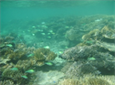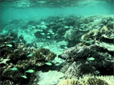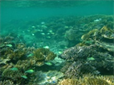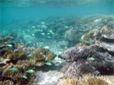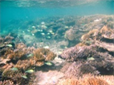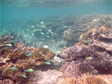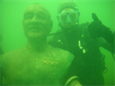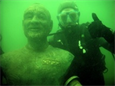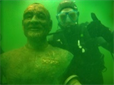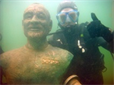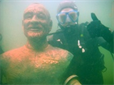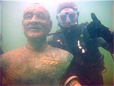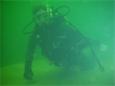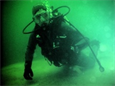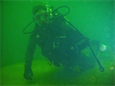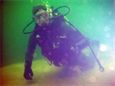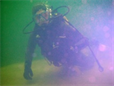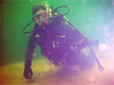PCQI ( a , b ) = q i ( a , b ) ⋅ q c ( a , b ) ⋅ q s ( a , b ) (12)

Sharpness = ∑ ( I ( x + 2 , y ) − I ( x , y ) ) 2 + ( I ( x , y + 2 ) − I ( x , y ) ) 2 (13)

Evaluation index of underwater image enhancement result

HE方法1.0482.0401.239
DCP方法0.9991.4240.985
RCP方法1.0903.1511.536
NUDCP方法1.0833.2141.560

5. 结束语